# How to use the MDETERM Function in Excel

In this article, we will learn about how to use the MDETERM function in Excel.

Determinant of a square matrix is a number useful in calculating system of linear equation or calculating the inverse of a matrix.

A Square matrix is matrix which have equal number of rows and columns. Below here are some examples of square matrices.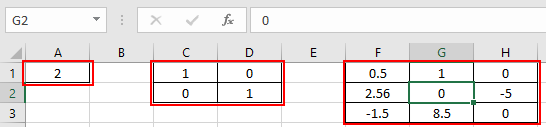First is a square matrix of 1 x 1. Second is square matrix of 2 x 2. Third matrix is of 3 x 3.

Determinant of a matrix is calculated using the below shown formula.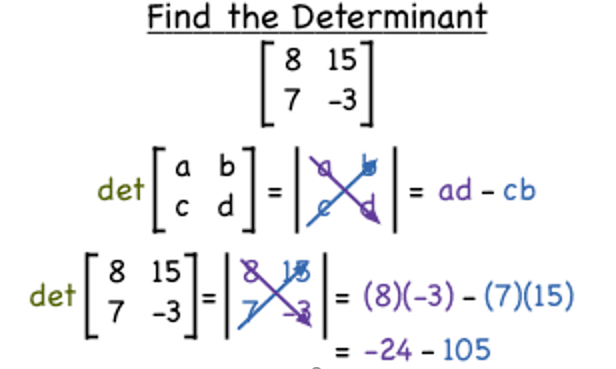There’s another example shown below for understanding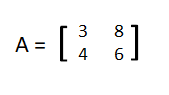| A | = 3 * 6 - 4 * 8
| A | = - 14
The MDETERM function returns a number representing the determinant of the given input matrix.
Syntax:

=MDETERM(array)

Array : numbers given as cell reference or directly using brackets and semi - colons.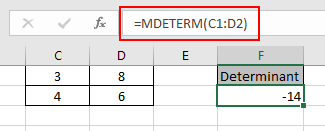The determinant of the above shown matrix is -14.
Let’s understand this function using it in an example.

Here we have 1 x 1 matrix. We need to calculate the determinant of the below shown 1 x 1 matrix.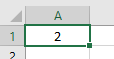Use the formula:

=MDETERM (A1)

A2 : array of a number.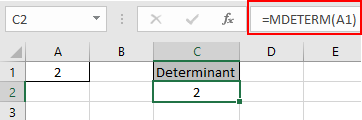Determinant of the number 2 comes out to be 2. So the determinant of 1 x 1 having one number is the number itself.
Now we will calculate the determinant of the 2 x 2 unit matrix shown below.

A unit or identity matrix is whose all diagonal numbers are 1 and all other numbers are zero.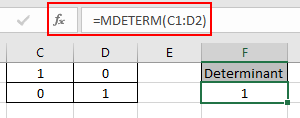Determinant of a unit matrix is the unit value 1.

array to the MDETERM function is provided as cell reference.

Now we will calculate the determinant of the 3 x 3 matrix shown below.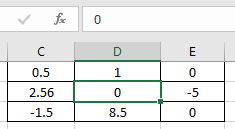Use the formula:

=MDETERM(C1:E3)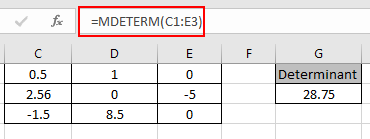As you can see here the MDETERM representation of the numbers from the numbers.

All the above example shown are used via giving argument to the function as cell reference.

you could get the determinant of the matrix providing the array directly to the function

Use the formula:

=MDETERM ( { 3, 4; 2, 3 } )

Values along the columns are separated by commas and values in different rows are separated by semi - colons.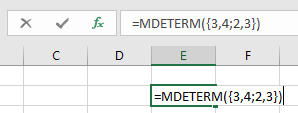Press Enter to view the result.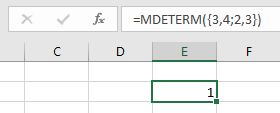The determinant of the matrix is 1. But it doesn’t mean this is a unit or identity matrix.

Notes:

1. The function returns the #VALUE! error if there is any non - numeric value given as argument to the function.
2. The matrix must be a square matrix i.e. number of rows equal to the number of columns of the matrix or otherwise the function returns the #VALUE! error.

Hope you understood how to use MDETERM function and referring cell in Excel. Explore more articles on Excel mathematical conversion functions here. Please feel free to state your query or feedback for the above article.

Related Articles

How to use the MINVERSE Function in Excel

How to use the MUNIT Function in Excel

Find the nth root of a number

Calculate Weighted Average

Ignore zero in the Average of numbers

Popular Articles

50 Excel Shortcuts to Increase Your Productivity

How to use the VLOOKUP Function in Excel

How to use the COUNTIF in Excel 2016

## Comments

1.REZA HASHEMIAN

I need to calculate the determinant of a matrix that is constructed from n arbitrary rows and n arbitrary columns of a bigger matrix. How can I do this with Excel? Thanks.

•You can do this using semicolon and curly braces. For example if you want to get the determinant of rows A1:B5 and columns D5:E9 then use this type of formula `=MDETERM({A1:B5;D5:E9})`

Terms and Conditions of use

The applications/code on this site are distributed as is and without warranties or liability. In no event shall the owner of the copyrights, or the authors of the applications/code be liable for any loss of profit, any problems or any damage resulting from the use or evaluation of the applications/code.